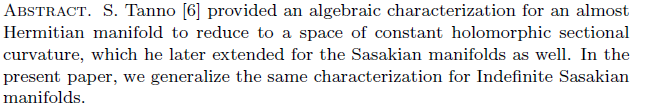Research Article

### Constancy of ϕ-Holomorphic Sectional Curvature for Indefinite Sasakian Manifolds

Year 2009, Volume 2, Issue 1, 34 - 40, 30.04.2009

### Abstract### References

•  Barros, M. and Remero, A., Indefinite Kahler manifolds, Math. Ann. 266(1982), 55-62.
•  Dajczer, M. and Nomizu, K., On sectional curvatures of indefinite metrics II ,Math. Ann. 247(1980), 279-282.
•  Graves, L. and Nomizu, K., On sectional curvature of indefinite metric I, Math. Ann. 232(1978), 267-272.
•  Ikawa, T. and Jun, J. B., On sectional curvatures of normal contact Lorentizian manifold, Korean J. Math. Sciences J. 4(1997), 27-33.
•  Nagaich, R. K., Constancy of holomorphic sectional curvature in inde¯nite almost Hermitian manifolds, Kodai Math. J. 16(1993), 327-331.
•  Tanno, S., Constancy of holomorphic sectional curvature in almost Hermitian manifolds, Kodai Math. Sem. Rep. 25(1973), 190-201.
Year 2009, Volume 2, Issue 1, 34 - 40, 30.04.2009

### References

•  Barros, M. and Remero, A., Indefinite Kahler manifolds, Math. Ann. 266(1982), 55-62.
•  Dajczer, M. and Nomizu, K., On sectional curvatures of indefinite metrics II ,Math. Ann. 247(1980), 279-282.
•  Graves, L. and Nomizu, K., On sectional curvature of indefinite metric I, Math. Ann. 232(1978), 267-272.
•  Ikawa, T. and Jun, J. B., On sectional curvatures of normal contact Lorentizian manifold, Korean J. Math. Sciences J. 4(1997), 27-33.
•  Nagaich, R. K., Constancy of holomorphic sectional curvature in inde¯nite almost Hermitian manifolds, Kodai Math. J. 16(1993), 327-331.
•  Tanno, S., Constancy of holomorphic sectional curvature in almost Hermitian manifolds, Kodai Math. Sem. Rep. 25(1973), 190-201.

### Details

Primary Language English Research Article Rachna RANİ This is me R. K. NAGAİCH This is me April 30, 2009 Year 2009, Volume 2, Issue 1

### Cite

 Bibtex @research article { iejg584310, journal = {International Electronic Journal of Geometry}, eissn = {1307-5624}, address = {}, publisher = {Kazım İLARSLAN}, year = {2009}, volume = {2}, number = {1}, pages = {34 - 40}, title = {Constancy of ϕ-Holomorphic Sectional Curvature for Indefinite Sasakian Manifolds}, key = {cite}, author = {Kumar, Rakesh and Rani, Rachna and Nagaich, R. K.} } APA Kumar, R. , Rani, R. & Nagaich, R. K. (2009). Constancy of ϕ-Holomorphic Sectional Curvature for Indefinite Sasakian Manifolds . International Electronic Journal of Geometry , 2 (1) , 34-40 . Retrieved from https://dergipark.org.tr/en/pub/iejg/issue/46545/584310 MLA Kumar, R. , Rani, R. , Nagaich, R. K. "Constancy of ϕ-Holomorphic Sectional Curvature for Indefinite Sasakian Manifolds" . International Electronic Journal of Geometry 2 (2009 ): 34-40 Chicago Kumar, R. , Rani, R. , Nagaich, R. K. "Constancy of ϕ-Holomorphic Sectional Curvature for Indefinite Sasakian Manifolds". International Electronic Journal of Geometry 2 (2009 ): 34-40 RIS TY - JOUR T1 - Constancy of ϕ-Holomorphic Sectional Curvature for Indefinite Sasakian Manifolds AU - RakeshKumar, RachnaRani, R. K.Nagaich Y1 - 2009 PY - 2009 N1 - DO - T2 - International Electronic Journal of Geometry JF - Journal JO - JOR SP - 34 EP - 40 VL - 2 IS - 1 SN - -1307-5624 M3 - UR - Y2 - 2022 ER - EndNote %0 International Electronic Journal of Geometry Constancy of ϕ-Holomorphic Sectional Curvature for Indefinite Sasakian Manifolds %A Rakesh Kumar , Rachna Rani , R. K. Nagaich %T Constancy of ϕ-Holomorphic Sectional Curvature for Indefinite Sasakian Manifolds %D 2009 %J International Electronic Journal of Geometry %P -1307-5624 %V 2 %N 1 %R %U ISNAD Kumar, Rakesh , Rani, Rachna , Nagaich, R. K. . "Constancy of ϕ-Holomorphic Sectional Curvature for Indefinite Sasakian Manifolds". International Electronic Journal of Geometry 2 / 1 (April 2009): 34-40 . AMA Kumar R. , Rani R. , Nagaich R. K. Constancy of ϕ-Holomorphic Sectional Curvature for Indefinite Sasakian Manifolds. Int. Electron. J. Geom.. 2009; 2(1): 34-40. Vancouver Kumar R. , Rani R. , Nagaich R. K. Constancy of ϕ-Holomorphic Sectional Curvature for Indefinite Sasakian Manifolds. International Electronic Journal of Geometry. 2009; 2(1): 34-40. IEEE R. Kumar , R. Rani and R. K. Nagaich , "Constancy of ϕ-Holomorphic Sectional Curvature for Indefinite Sasakian Manifolds", International Electronic Journal of Geometry, vol. 2, no. 1, pp. 34-40, Apr. 2009